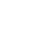# Invert a Binary TreeAlex Woods

July 29, 2021

In an inverted binary tree, each node in the original is swapped with the corresponding node on the other side.

You can also think of it as rotating the tree on an axis right down the middle.

Example:Here's the same tree, inverted.Like most tree problems, it's best handled recursively. You just

• Swap
• Recurse to the left child
• Recurse to the right child
``````def invertBinaryTree(tree):
# This is why I use Python for stuff like this
tree.left,tree.right = tree.right,tree.left

if (tree.left is not None):
invertBinaryTree(tree.left)
if (tree.right is not None):
invertBinaryTree(tree.right)

class BinaryTree:
def __init__(self, value):
self.value = value
self.left = None
self.right = None
``````

It's surprisingly simple.

Want to know when I write a new article?

Get new posts in your inbox